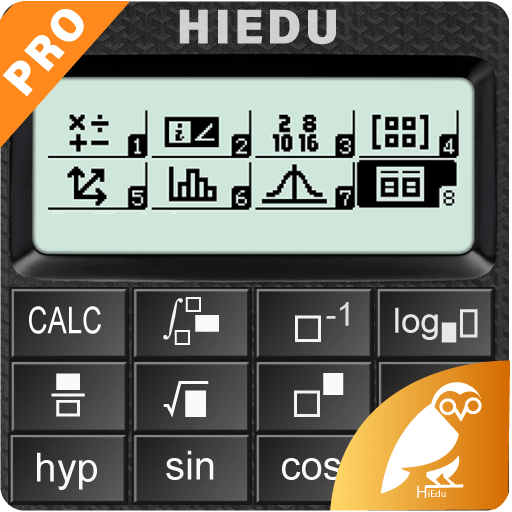HiEdu Scientific Calculator He-580 Pro V1.1.6
Visits: 9 | Updated: 11-Apr-2021
Download Categories
Share Download
Friends & Affiliates
Popular Searches
Apps4all Information
HiEdu Scientific Calculator He-580 Pro V1.1.6
HiEdu Scientific Calculator He-580 Pro V1.1.6 returned downloads at this site:

Found 1 download for HiEdu Scientific Calculator He-580 Pro V1.1.6
HiEdu Scientific Calculator He-580 Pro V1.1.6
Download Information & File Host Links
Category: Mobile | Date: 06-Apr-2021HiEdu Scientific Calculator He-580 Pro v1.1.6 | Requirements: Android 4.1+ | File size: 5,6 MB

Key features:

Scientific Calc:
It is a scientific calculator supports most of the features of real calculators.
Natural Display makes it possible to input and display fractions and certain functions (log, x2, x3, x^, ), ", x-1, 10^, e^ , d/dx, S, Abs ...) just as they are written in your textbook.
Undo when you miss a mistake.
Save history, Select a calculation in list history and edit it.
Create favorite calculations that make calculations faster.

Standard Calc:
This is a perfect tool for daily calculations. With memory functions similar to a small handheld calculator
Save history, Select a calculation in list history and edit it.

Mathematics formulas:
This app has 1000+ math formula and more to come.
Now no need to make paper notes to remember mathematics formulas just have this app put all the formulas on your favourite phones.
you'll find formulas very simply explained in app with necessary figures will help you to understand very easily.

Physics formulas:
The application is a physical handbook. Contains most physical formulas for students and undergraduate.help users quickly refer to any Physics formulas for their study and work.This app displays most popular as well as advanced formulas in seven categories:
Mechanics
Electricity
Thermal physics
Periodic motion
Optics
Atomic physics
Constants

Chemical reactions:
Allows to discover chemical reactions and to solve the chemical equations with one and several unknown variables.

Programmers:
Can convert numbers between different number bases (2/8/10/16).
Display shows numbers in binary, hexadecimal, octal and decimal.
Input can be in binary, hexadecimal, octal or decimal.

Graphing calc:
Easily graph functions, solve equations, find special points of functions.

Solve the equations:
Linear equation for degree one,quadratic equation for degree two,cubic equation for degree three, System of linear equations.

Converter:
Available unit conversions include:
Currency (US dollar, CDN dollar, pound, peso, etc)
Temperature (celsius, fahrenheit, kelvin, etc)
Length (kilometer, miles, meter, yard, feet, etc)
Mass/Weight (kilogram, pound, ounce, ton, stone, etc)
Speed (km/h, mph, knot, etc)
Area (square kilometer, square mile, hectare, acre, etc)
Cooking Volume (teaspoon, tablespoon, cup, pint, quart, ounce, etc)
Pressure (kilopascal, bar, PSI, etc)
Power (watt, kilowatt, horsepower, etc)
Energy (joule, calorie, BTU, etc)
Time (year, month, day, hour, second, etc)
Fuel Consumption (miles per gallon, liters per 100km, etc)
Digital Storage (bit, byte, megabytes, gigabytes, etc)

Display:
The layout of the buttons in the this app is scientifically rigorous, which makes entering mathematical operations as simple and convenient as possible.
Numeric buttons and functions in the our application are beautifully designed, clear, resistant to glare, blurry and eyestrain.
There are many beautiful themes with different styles.

WHAT'S NEW
1.1.6

Add chat function: Ask questions with our experts.
Add tax function: Use tax calculation.

Homepage
``https://anonymz.com/...80pro&hl=en``https://rapidgator.net/file/e6f1ddef931b6ce42692736ac2b3df8f/HiEdu_Scientific_Calculator_Pro_v1.1.6.rar.html

https://nitroflare.com/view/C7CE1F324957CB4/HiEdu_Scientific_Calculator_Pro_v1.1.6.rar